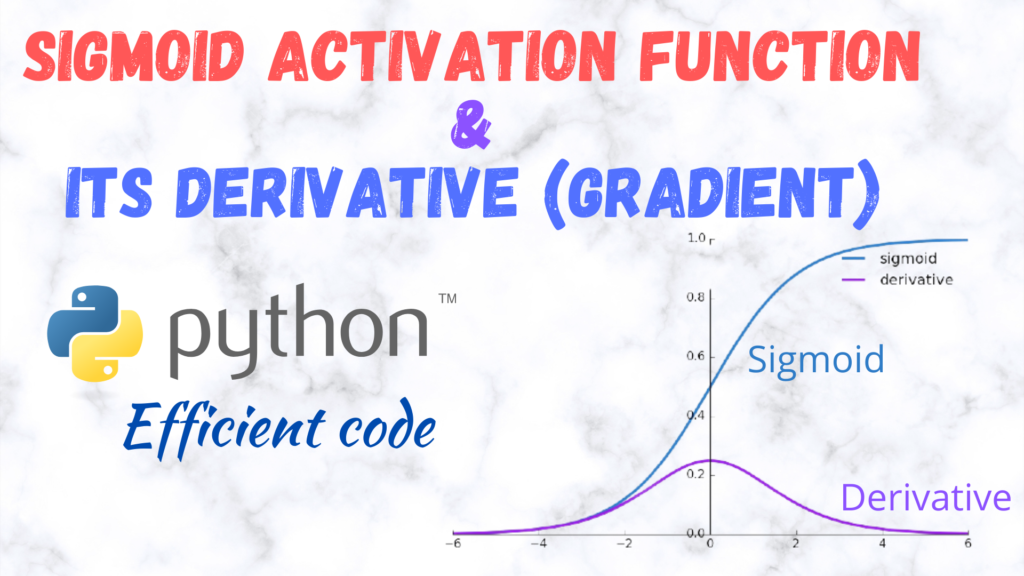Efficient implementation of Sigmoid activation function and its Derivative (gradient) in PythonThe mathematical definition of the Sigmoid activation function is$\sigma(x)= \frac{1}{1+\exp(-x)}$

and its derivative is$\sigma'(x)=\frac{\exp{(-x)}}{\left(1+\exp{(-x)}\right)^{2}}$

The Sigmoid function and its derivative for a batch of inputs (a 2D array with nRows=nSamples and nColumns=nNodes) can be implemented in the following manner:
Sigmoid simplest implementation

import numpy as np
def Sigmoid(x):
return 1/(1+np.exp(-x))

Sigmoid derivative simplest implementation

import numpy as np
return np.exp(-x)/(np.exp(-x)+1)**2

However, these implementations can be further accelerated (sped-up) by using Numba (https://numba.pydata.org/). Numba is a Just-in-time (JIT) compiler that

translates a subset of Python and NumPy code into fast machine code.

To use numba, install it as:

pip install numba

Also, make sure that your numpy is compatible with Numba or not, although usually pip takes care of that. You can get the info here: https://pypi.org/project/numba/

Accelerating the above functions using Numba is quite simple. Just modify them in the following manner:

Sigmoid NUMBA implementation

from numba import njit
@njit(cache=True,fastmath=True)
def Sigmoid(x):
return 1/(1+np.exp(-x))

Sigmoid derivative NUMBA implementation

from numba import njit
@njit(cache=True,fastmath=True)
e_x = np.exp(-x)
return e_x/(e_x+1)**2

While the implementations above seem simple and fast, they suffer from a big problem, i.e., they are susceptible to overflow or underflow.
To avoid under/overflow use the following alternative efinitions:

Sigmoid stable NumPy implementation 1

def Sigmoid(x): # Also known as logistic/soft step or even expit in scipy.special
# Alternative 1 (Doesn't work with Numba as boolean masking (fancy indexing) is not supported for 2D arrays -https://stackoverflow.com/questions/57915632/numba-nopython-mode-cannot-accept-2-d-boolean-indexing )
# Hao Peng's answer from here https://stackoverflow.com/questions/51976461/optimal-way-of-defining-a-numerically-stable-sigmoid-function-for-a-list-in-pyth
# Boolean array inversion is faster than another comparison
z = np.zeros_like(x)
top = np.ones_like(x)

Sigmoid stable NumPy implementation 2

def Sigmoid(x): # Also known as logistic/soft step or even expit in scipy.special
# Alternative 2 (Quite slow on CPU but fast enough on GPU)
# Neil G's answer from here https://stackoverflow.com/questions/3985619/how-to-calculate-a-logistic-sigmoid-function-in-python

Sigmoid stable NUMBA implementation 3

@njit(cache=True,fastmath=True, parallel=True)
def Sigmoid(x): # Also known as logistic/soft step or even expit in scipy.special
# Hao Peng's answer from here https://stackoverflow.com/questions/51976461/optimal-way-of-defining-a-numerically-stable-sigmoid-function-for-a-list-in-pyth
# Works only for 2D arrays
output = np.zeros((x.shape,x.shape),dtype=x.dtype)
for i in prange(x.shape):
for j in range(x.shape):
x_val = x[i,j]
if x_val>=0:
output[i,j] = 1. / ( 1. + np.exp(-x_val) )
else:
e_x = np.exp(x_val)
output[i,j] = e_x / ( 1. + e_x )
return output

The last one based on Numba is quite fast and competitive with Tensorflow and PyTorch due to parallelization(https://github.com/manassharma07/crysx_nn/blob/main/benchmarks_tests/Performance_Activation_Functions_CPU.ipynb).

It is in fact also used in the CrysX-Neural Network library (crysx_nn)

Furthermore, the above implementations can be further accelerated using Cupy (CUDA), if using single precision (float32) is not a problem.

CuPy is an open-source array library for GPU-accelerated computing with Python. CuPy utilizes CUDA Toolkit libraries to make full use of the GPU architecture.

The Cupy implementations look as follows:

Sigmoid unstable Cupy implementation

import cupy as cp
def Sigmoid_cupy(x):
return 1/(1+cp.exp(-x))

Sigmoid stable Cupy implementation

def Sigmoid_cupy(x):

e_x = cp.exp(-x)
return e_x/(e_x+1.)**2

The above code is also used in the crysx_nn library.
To see how the crysx_nn implementations of Sigmoid compare with TensorFlow and PyTorch, click here.

I hope you found this information useful.

If you did, then don’t forget to check out my other posts on Machine Learning and efficient implementations of activation/loss functions in Python.

References

https://stackoverflow.com/questions/57915632/numba-nopython-mode-cannot-accept-2-d-boolean-indexing

https://stackoverflow.com/questions/51976461/optimal-way-of-defining-a-numerically-stable-sigmoid-function-for-a-list-in-pyth

https://stackoverflow.com/questions/3985619/how-to-calculate-a-logistic-sigmoid-function-in-python[wpedon id="7041" align="center"]